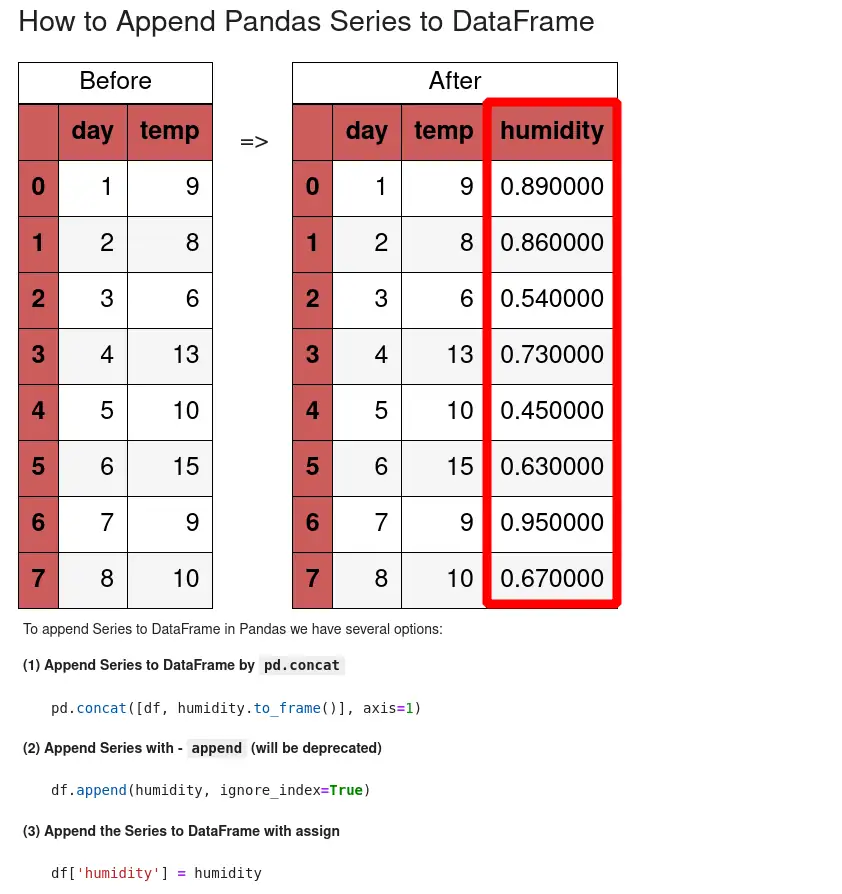To append Series to DataFrame in Pandas we have several options:

(1) Append Series to DataFrame by `pd.concat`

``````pd.concat([df, humidity.to_frame()], axis=1)
``````

(2) Append Series with - `append` (will be deprecated)

``````df.append(humidity, ignore_index=True)
``````

(3) Append the Series to DataFrame with assign

``````df['humidity'] = humidity
``````

## Setup

In the examples, we'll use the following setup, which consists of 1 DataFrame and 2 Series:

``````import pandas as pd
import matplotlib.pyplot as plt

data={'day': [1, 2, 3, 4, 5],
'temp': [9, 8, 6, 13, 10]}

df = pd.DataFrame(data)
``````

data is:

day temp
0 1 9
1 2 8
2 3 6
3 4 13
4 5 10

The series are:

``````ser_col = pd.Series(data=[0.89, 0.86, 0.54, 0.73, 0.45], name='humidity')
``````

which contains data for a new column:

``````0    0.89
1    0.86
2    0.54
3    0.73
4    0.45
Name: humidity, dtype: float64
``````

And a Series which contains data for a new row:

``````ser_row = pd.Series({'day': 6, 'temp': 15})
``````

result:

``````day      6
temp    15
dtype: int64
``````

## 1: Append Series to DataFrame - pd.concat as column

Let's start by appending Pandas Series to DataFrame by method `pd.concat()`.

We will add the Series to the DataFrame as a new column - this is possible by using parameter - `axis=1`:

``````pd.concat([df, ser_col.to_frame()], axis=1)
``````

This will append the Series as a new column to the DataFrame:

day temp humidity
0 1 9 0.89
1 2 8 0.86
2 3 6 0.54
3 4 13 0.73
4 5 10 0.45

## 2: Append Series to DataFrame - pd.concat as row

Next, we'll add the Series as a new row to a DataFrame. The method concat can be used once again:

``````pd.concat([df, ser_row.to_frame().T], ignore_index=True)
``````

We can ignore the index by using - `ignore_index=True`:

day temp
0 1 9
1 2 8
2 3 6
3 4 13
4 5 10
5 6 15

This is equivalent to `axis=0`## 3. Add Series to DataFrame with append

Let's see how to use the method `pd.append()` which will be deprecated in future. But it's still working and can be useful in some cases.

Pro Tip 1
Method pd.append is deprecated since version 1.4.0: Use concat() instead.

### Append Series as a row

To append Pandas Series as a new row to a DataFrame we can do:

``````df.append(ser_row,ignore_index=True)
``````

The new DataFrame is:

day temp
0 1 9
1 2 8
2 3 6
3 4 13
4 5 10
5 6 15

## 4. Add Series to DataFrame as column

Finally, let's take a look at a solution with assigning a new Series as a column in Pandas DataFrame. We can simply assign the Series to the DataFrame:

``````df['humidity'] = ser_col
``````

The result is the a new column:

day temp humidity
0 1 9 0.89
1 2 8 0.86
2 3 6 0.54
3 4 13 0.73
4 5 10 0.45
Pro Tip 1
Pandas Series can be considered as a DataFrame column - that's why we can add them to a DataFrame.

## Conclusion

In this article, we looked at different solutions for appending/adding a Series to DataFrame in Pandas. We focused on the most popular solutions with `pd.concat` but also covered a few alternatives.

We saw how to add a Series as a column or a row.